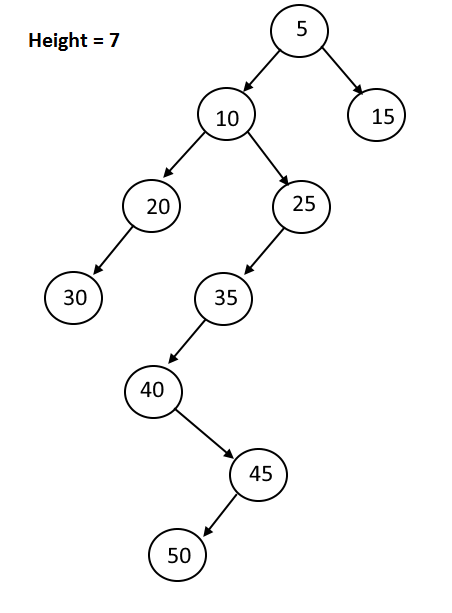Maximum Depth Of A Binary Tree

Posted: 12 Jan, 2021
Difficulty: Easy

PROBLEM STATEMENT

Depth of a binary tree is the same as its height. In simpler terms, you have to find the total number of nodes encountered while moving from the root node to the farthest leaf node, along the longest path of the binary tree.

Example:-If we are given the above binary tree as input then moving from root node(5) to the farthest leaf node(50), the path formed will be [ 5->10->25->35->40->45->50 ]. The total number of nodes encountered is 7, therefore the maximum depth of the binary tree is 7.
Input Format:
The first line of the input contains a single integer T, representing the number of test cases.

The first and only line of each test case contains the values of the nodes of the tree in the level order form ( -1 for NULL node) Refer to the example for further clarification.
Example:
Consider the following binary treeThe input of the tree shown in the above image will look like:

1 2 3 4 -1 5 6 -1 7 -1 -1 -1 -1 -1 -1

Explanation :
Level 1 :
The root node of the tree is 1

Level 2 :
Left child of 1 = 2
Right child of 1 = 3

Level 3 :
Left child of 2 = 4
Right child of 2 = null (-1)
Left child of 3 = 5
Right child of 3 = 6

Level 4 :
Left child of 4 = null (-1)
Right child of 4 = 7
Left child of 5 = null (-1)
Right child of 5 = null (-1)
Left child of 6 = null (-1)
Right child of 6 = null (-1)

Level 5 :
Left child of 7 = null (-1)
Right child of 7 = null (-1)

The first not-null node (of the previous level) is treated as the parent of the first two nodes of the current level. The second not-null node (of the previous level) is treated as the parent node for the next two nodes of the current level and so on. The input ends when all nodes at the last level are null (-1).
Output Format:
For each test case, print a single integer “N” representing the maximum depth of the input binary tree.
Note:
You do not need to print anything. It has already been taken care of. Just implement the given function.
Constraints:
1 <= T <= 100
1 <= N <= 3000
0 <= data <= 10^9

Time Limit: 1secApproach 1

The idea is to use DFS (Depth First Search) traversal and recursively find the maximum depths of the left subtree and right subtree, returning the max of both the values plus 1 (counting the root node) as our answer.

• We are given a function ‘findMaxDepth’ which takes the root node of the binary tree “ROOT”  as the parameter and returns an integer which is the maximum depth of the binary tree.
• First, we will take care of our base condition and check whether our ROOT node is NULL or not. If it is NULL, we will return 0, Implying that there is no node in the given tree and the height returned will be 0.
• Now, we will compute the maximum depth of both our left and the right subtree by recursively calling our function ‘findMaxDepth’ on the left child and the right child of the ‘ROOT’ node.
• Finally, we will find the maximum of both the maxDepths of left and right subtree. We will return the maximum depth plus 1 (counting the root node) as our final answer.